# Selina Solutions for Class 9 Physics Chapter 4 - Pressure in Fluids and Atmospheric Pressure

Selina solutions present students of class 9 with solutions to all the exercise questions provided in the ICSE Class 9 textbook in as per the ICSE exam. At BYJU’S, solutions are framed in the best way possible, effectively communicating concepts to students through various examples and diagrams to support solutions.

Solutions at BYJU’S infuses study sources by experts with extensive knowledge in their corresponding expertise thereby instilling a robust study source in the form of Selina solutions. Solutions are compiled considering the latest syllabus, covering the latest in the field of science and technology. All these considerations while preparing solutions, makes Selina solutions students’ favourite.

The concepts are clearly put across for students to enable self-study, thereby developing the analyzing capabilities of students and strengthening fundamentals which helps to better understand the concepts, its significance and its various applications. Students can access ICSE Class 9 Physics Selina Solutions of Chapter 4 by clicking mentioned below link.

### Access Answers of Physics Selina class 9 Chapter 4 – Pressure in Fluids and Atmospheric Pressure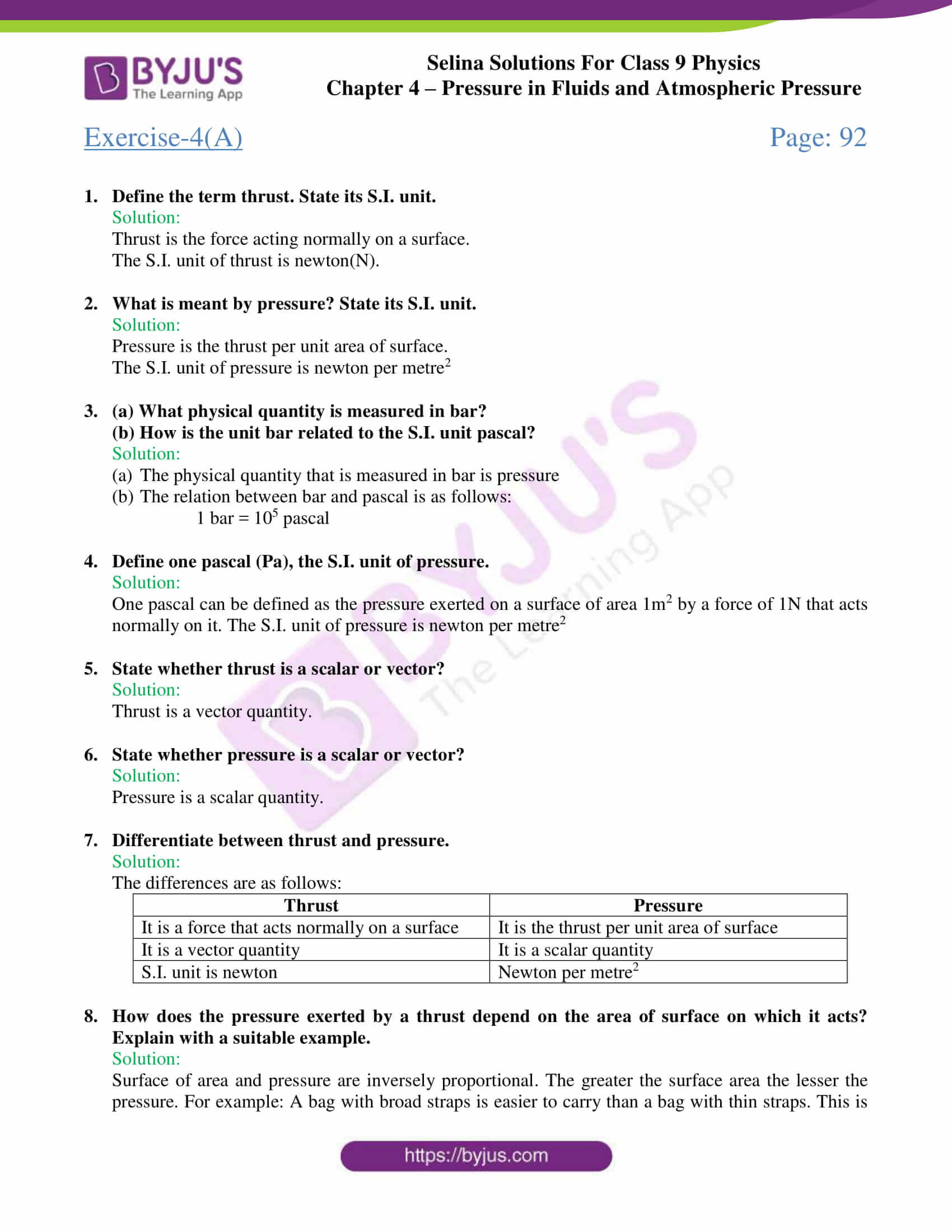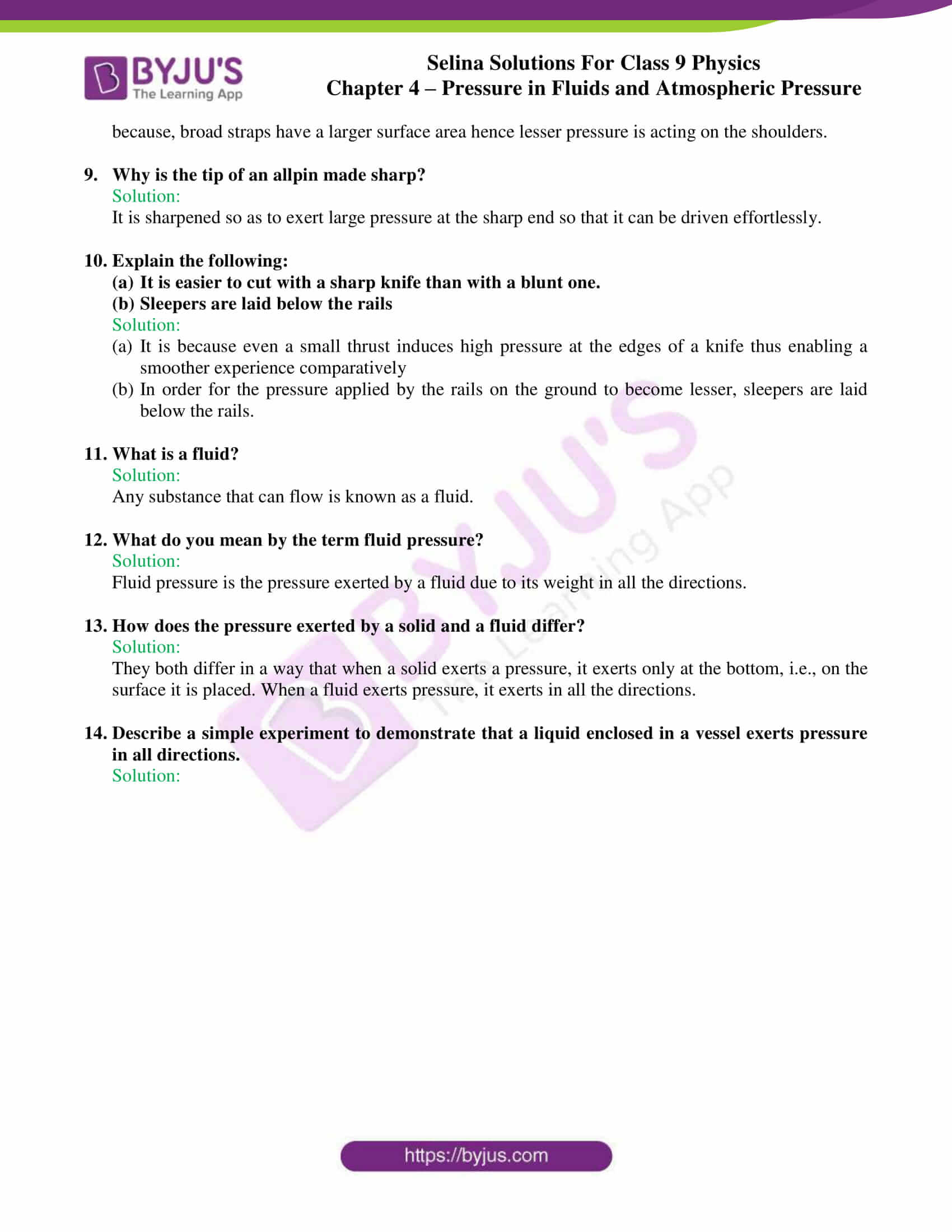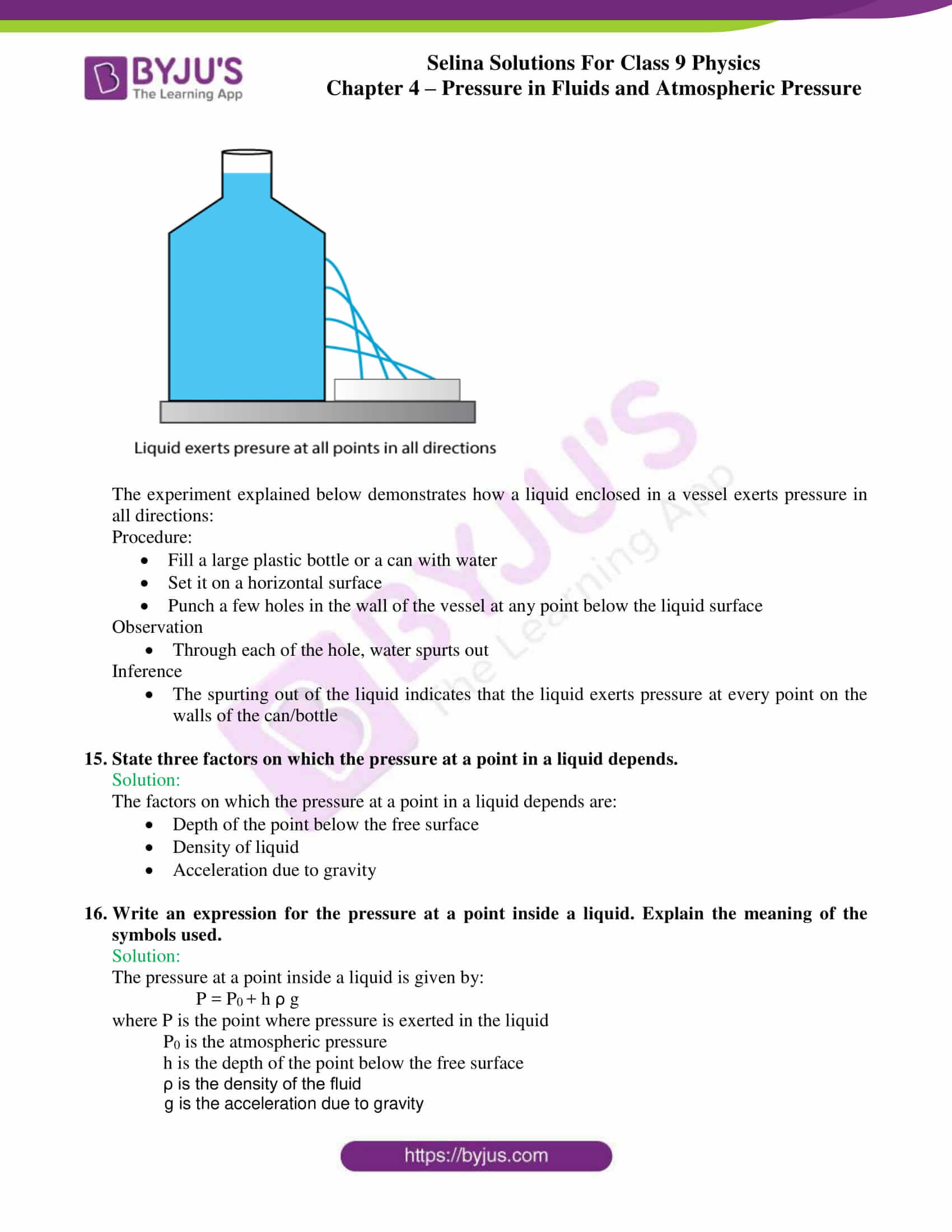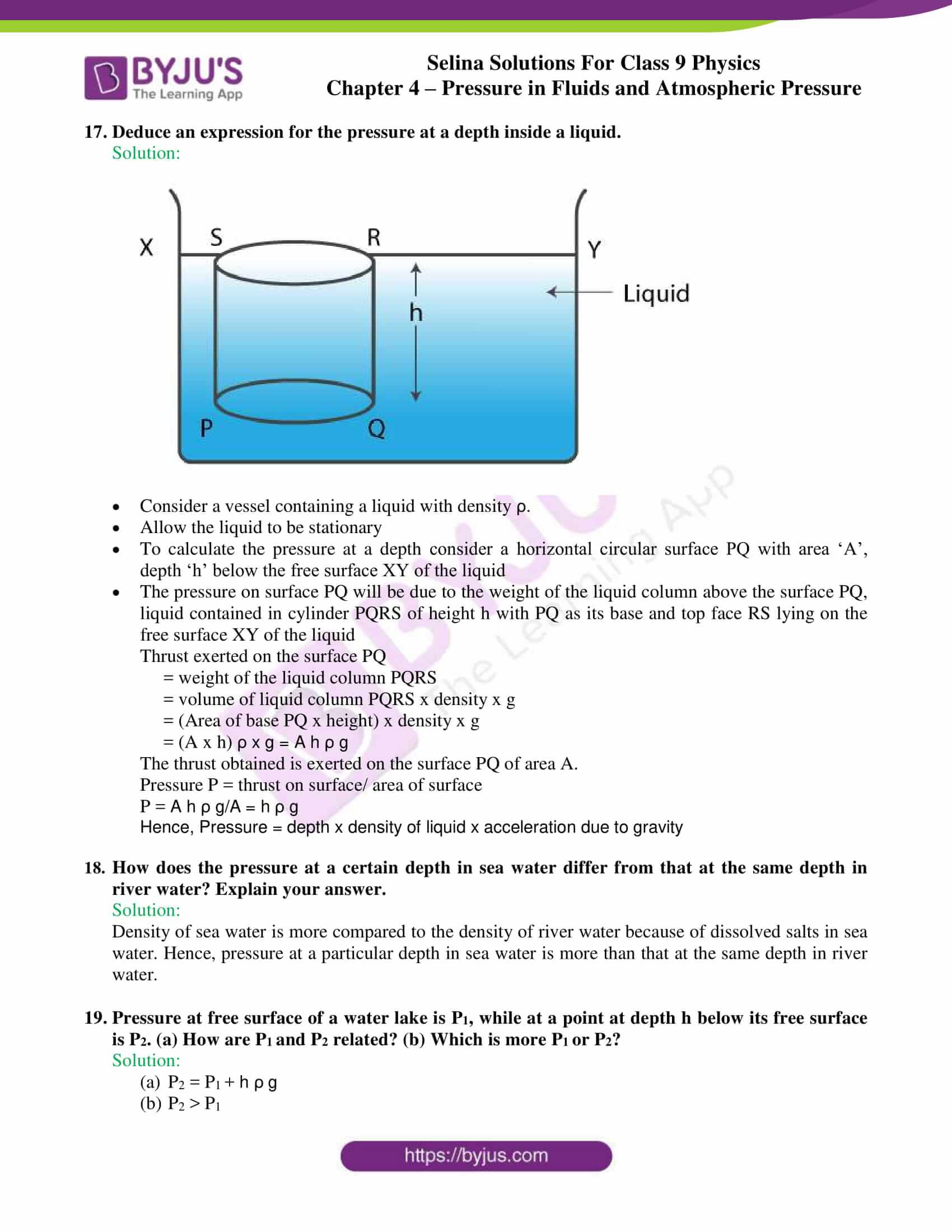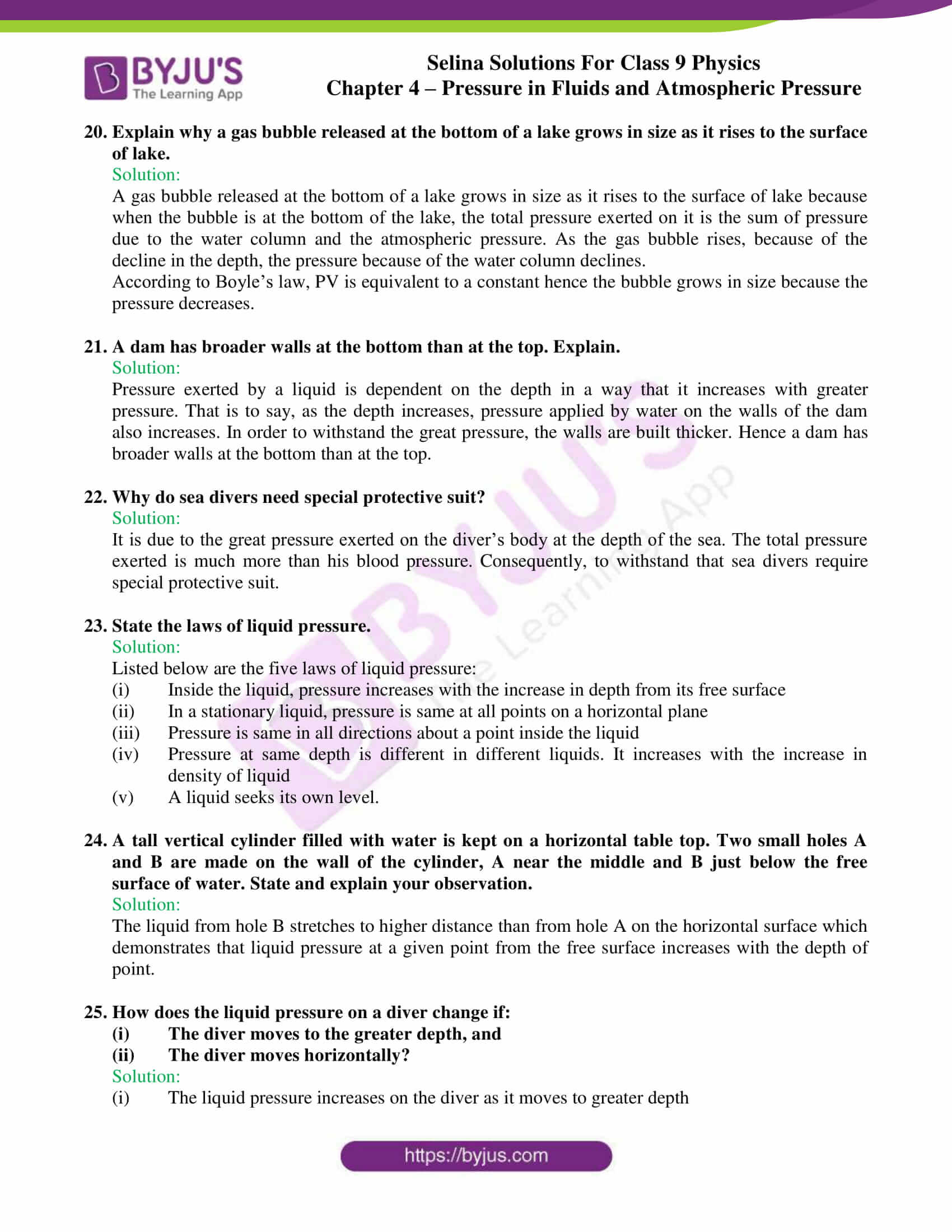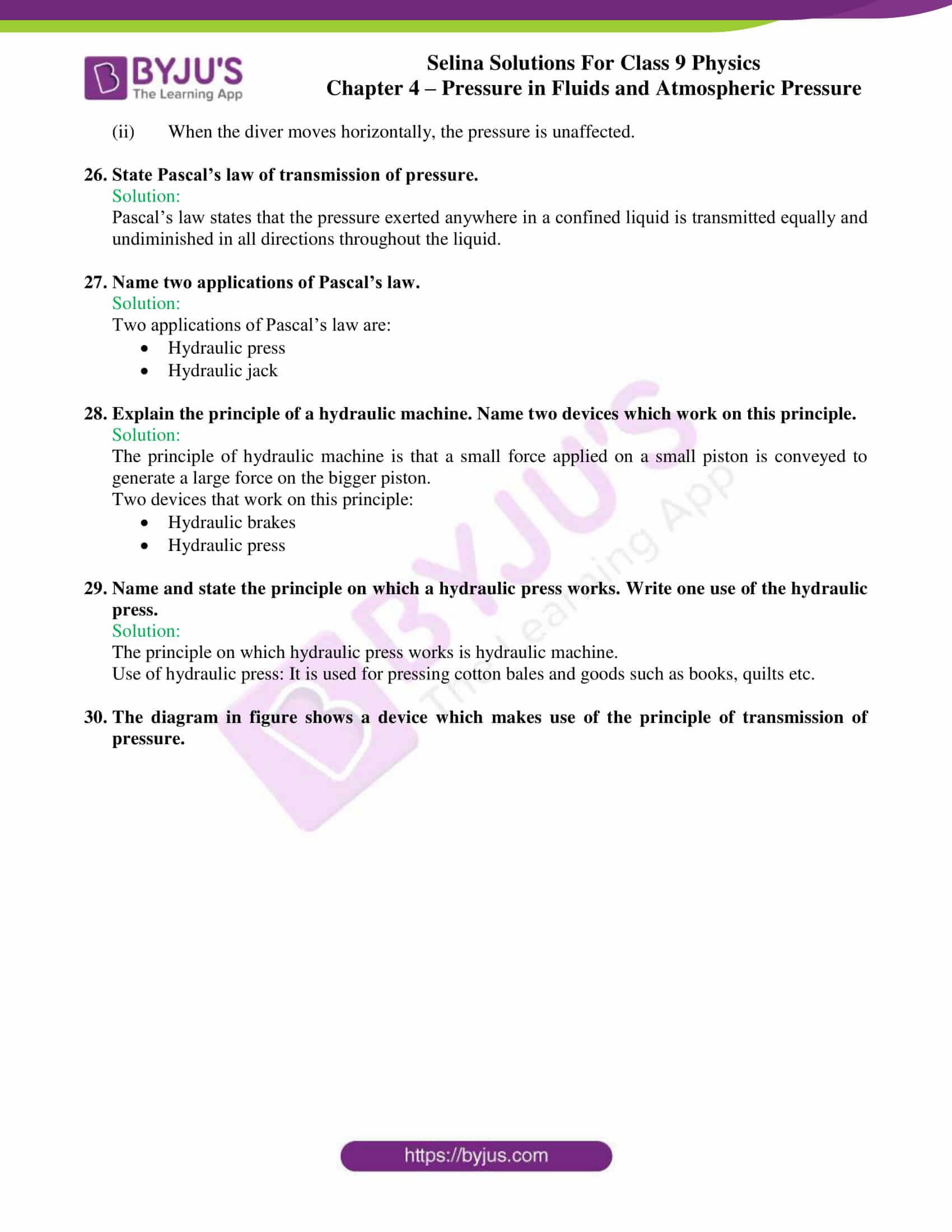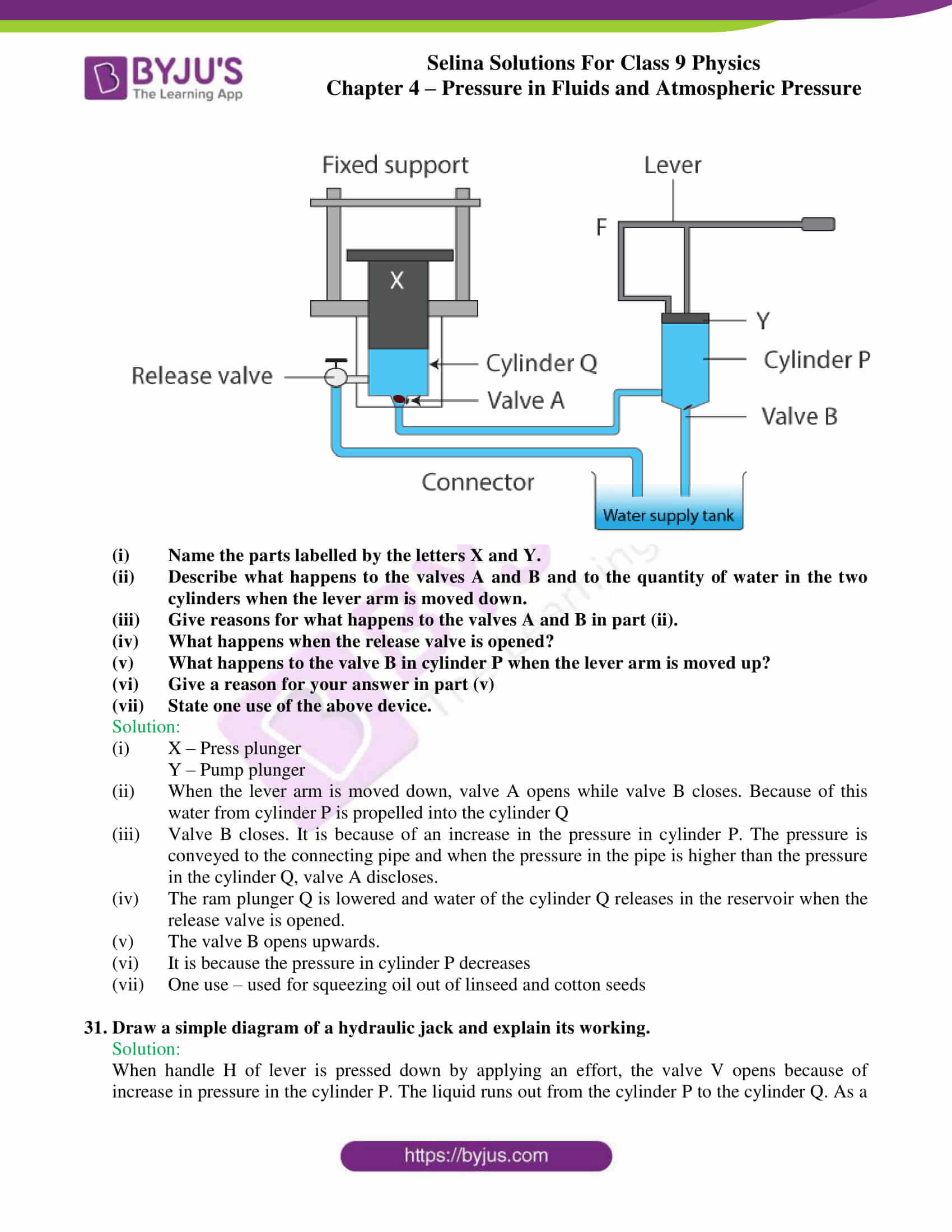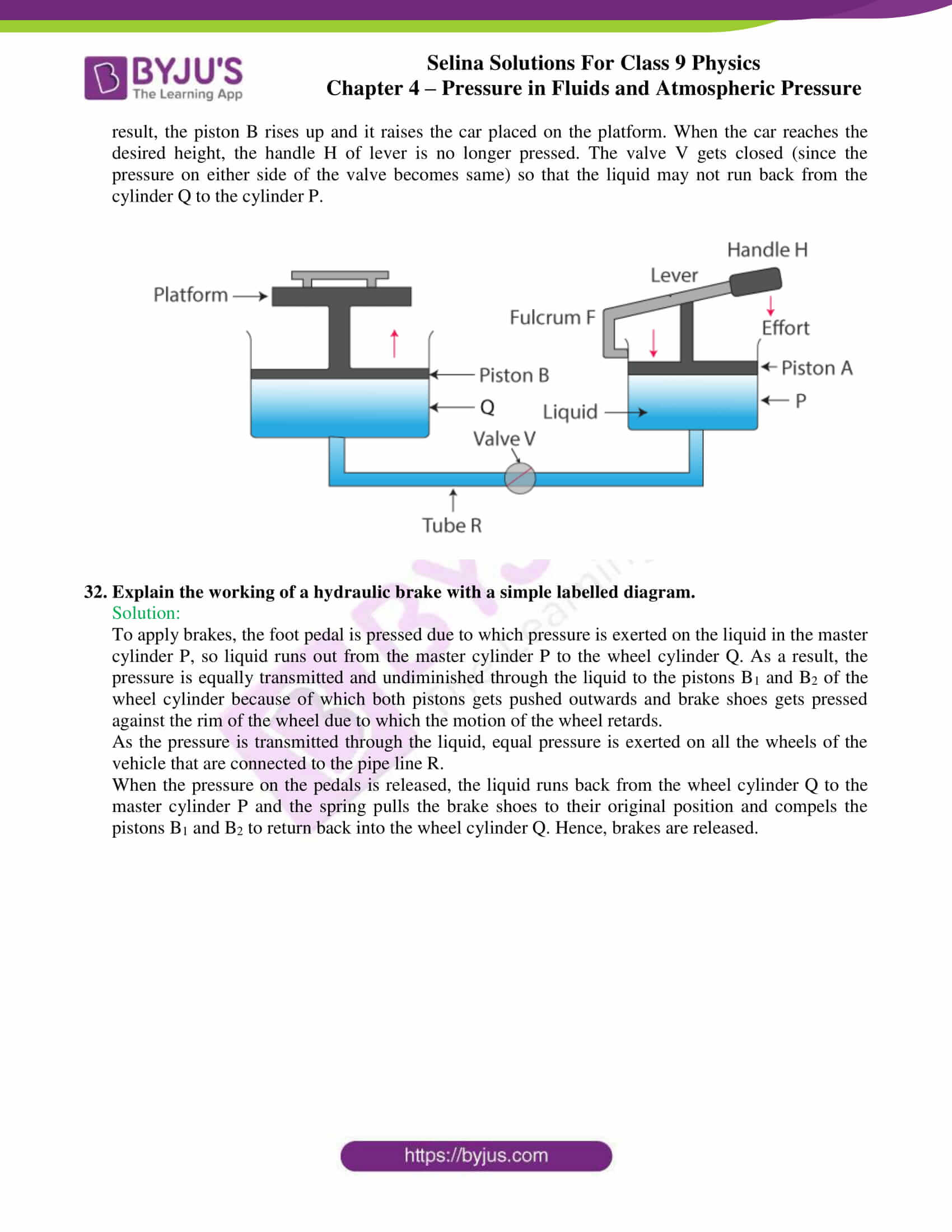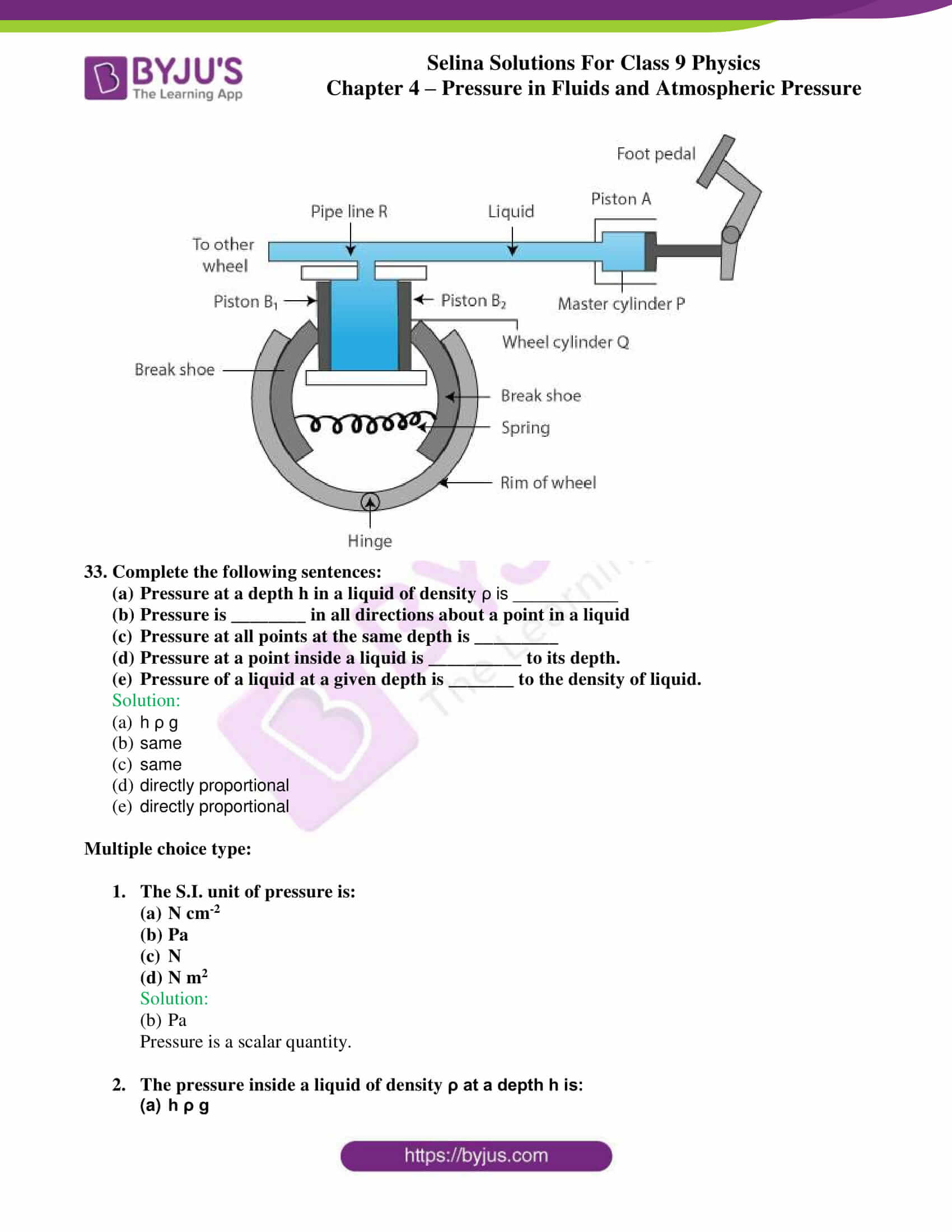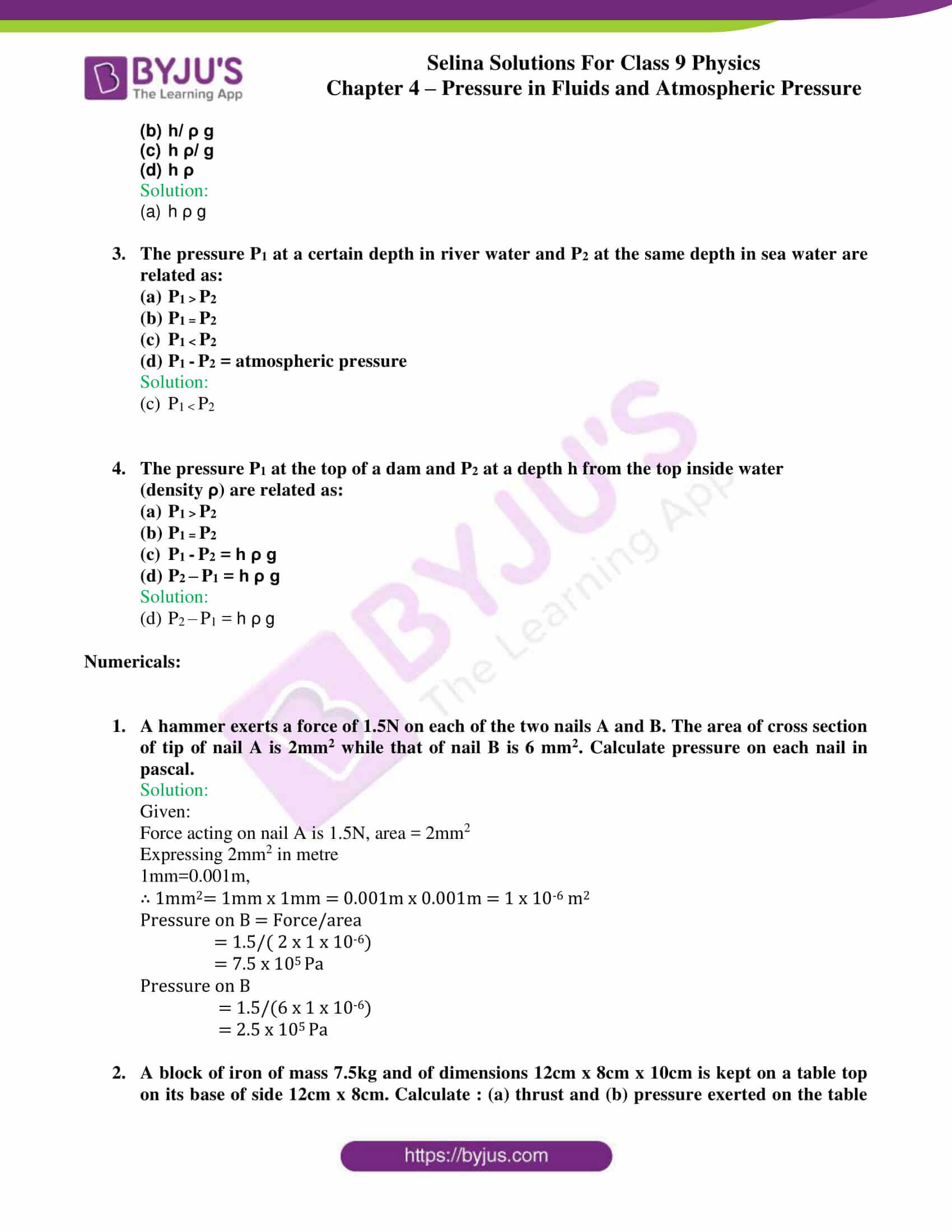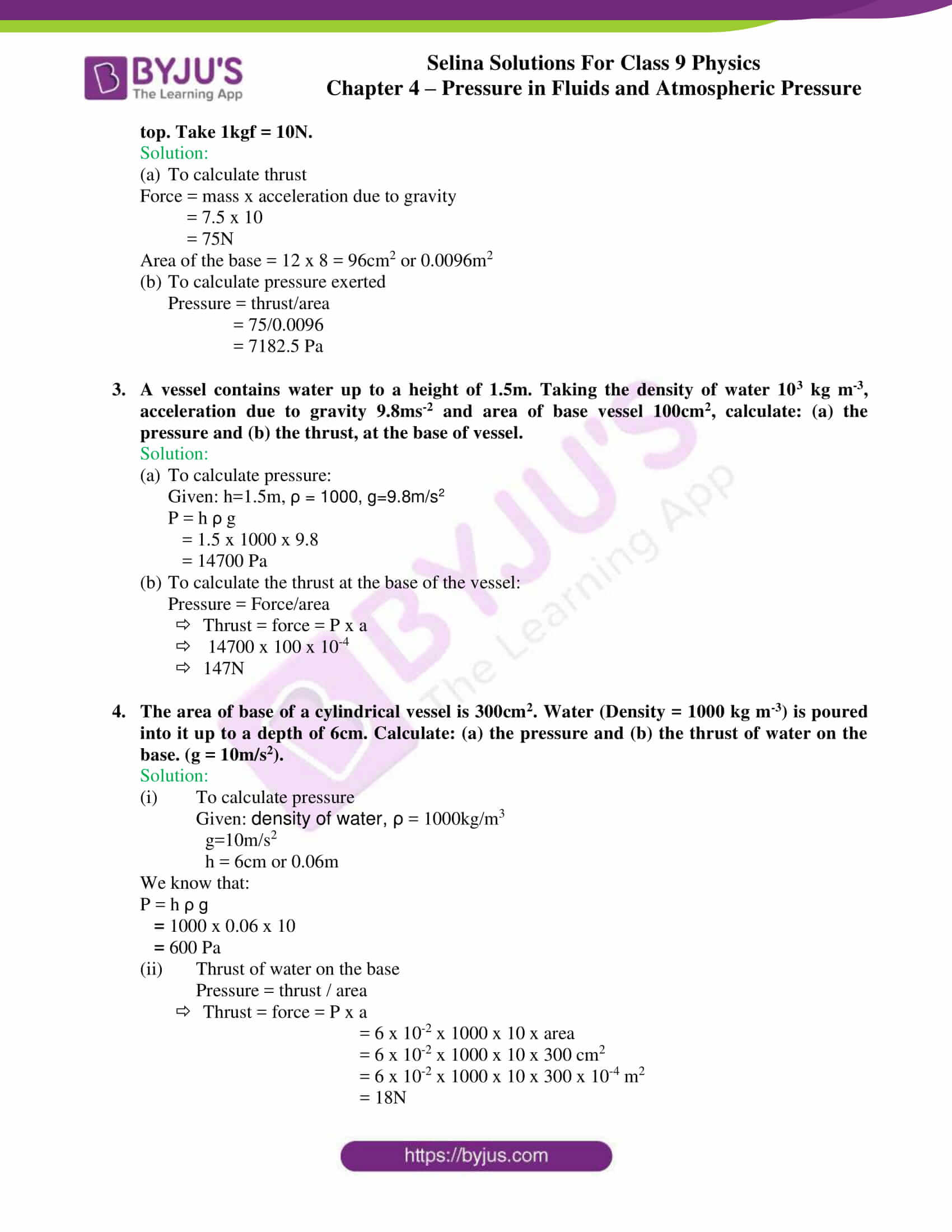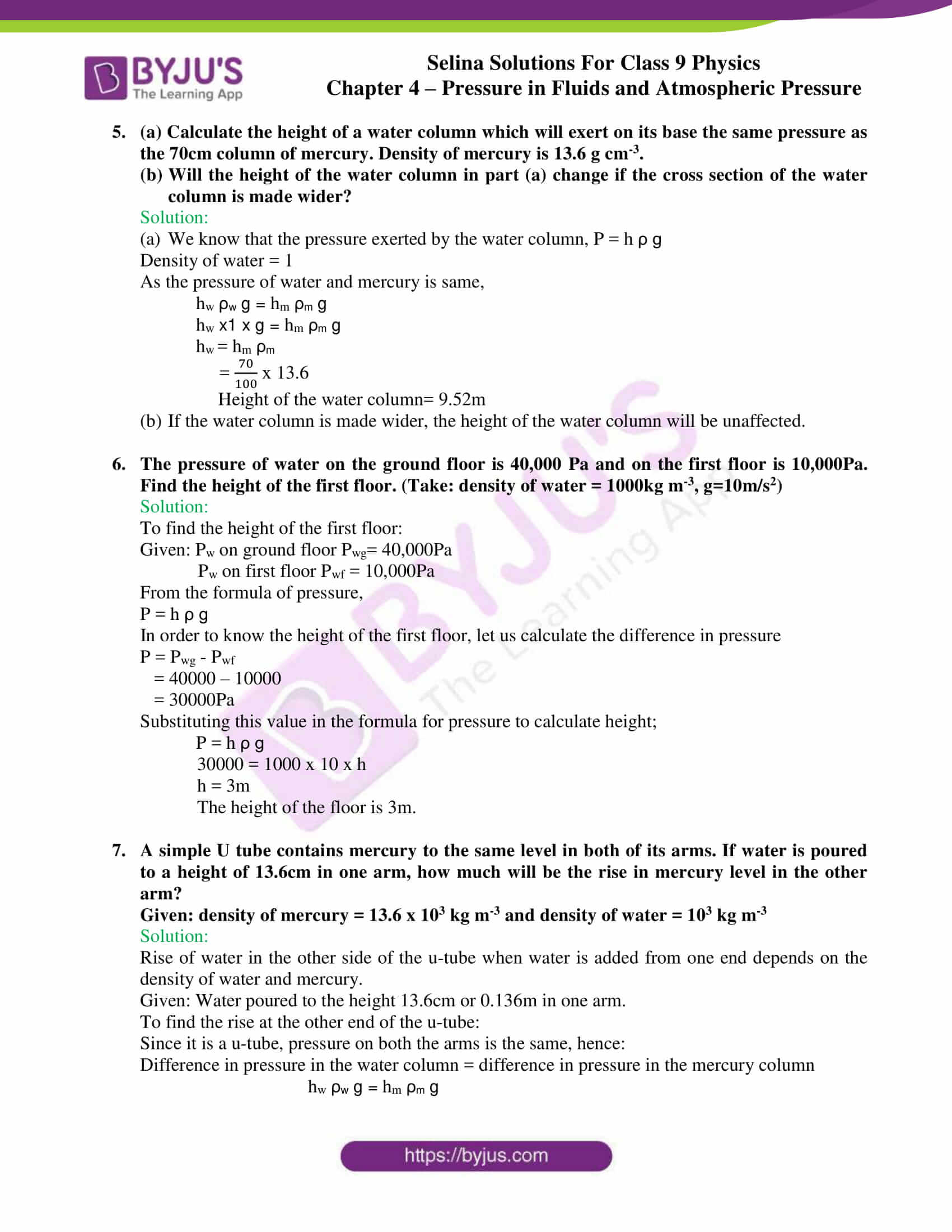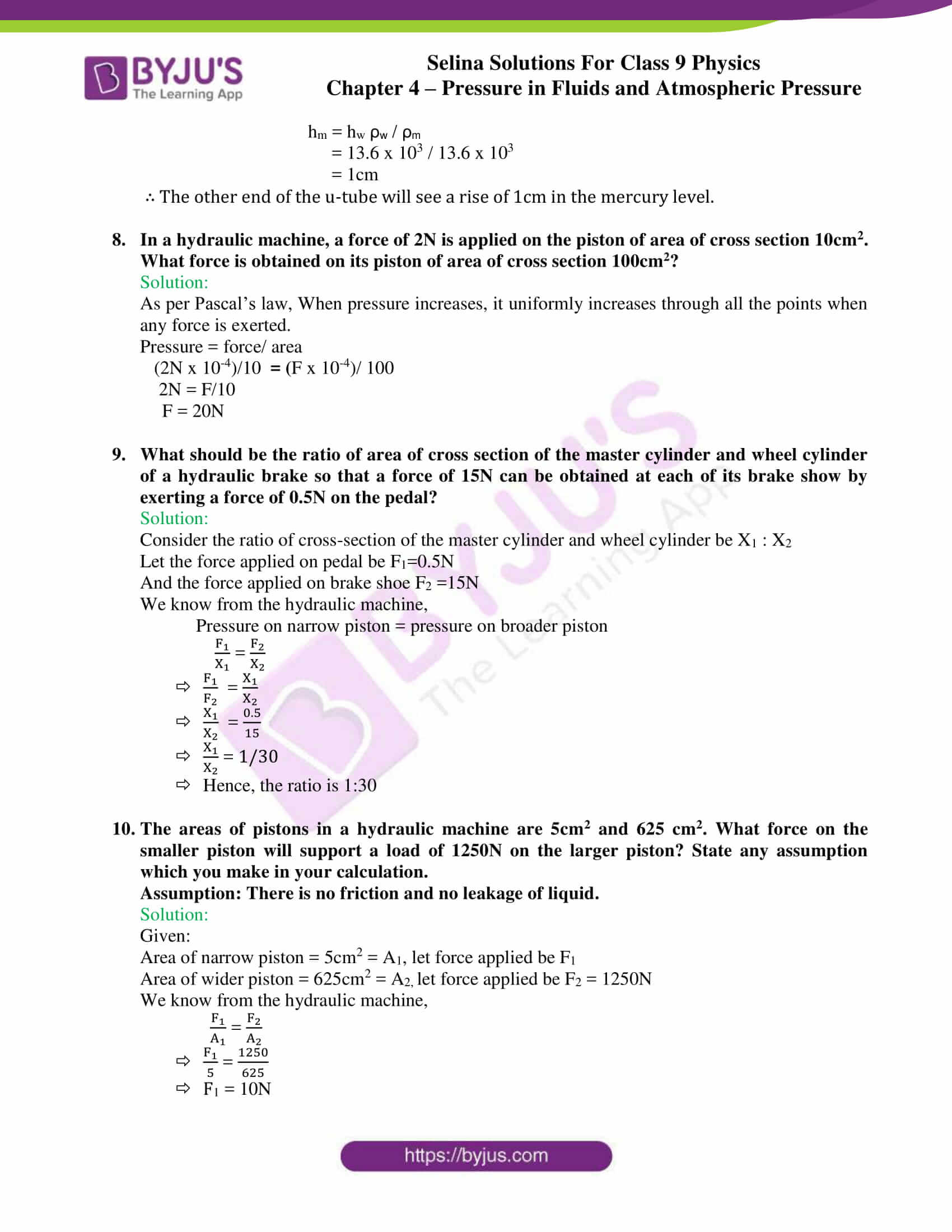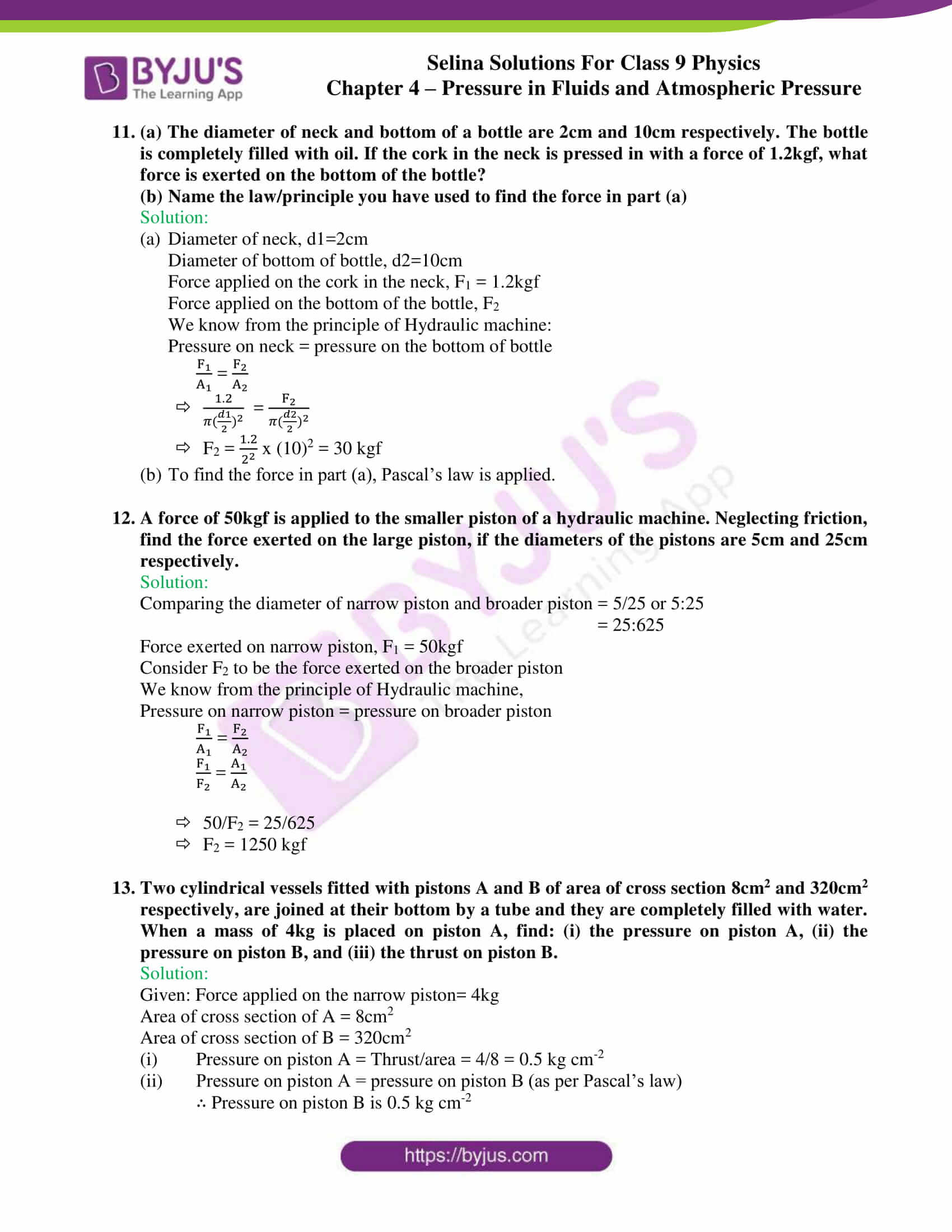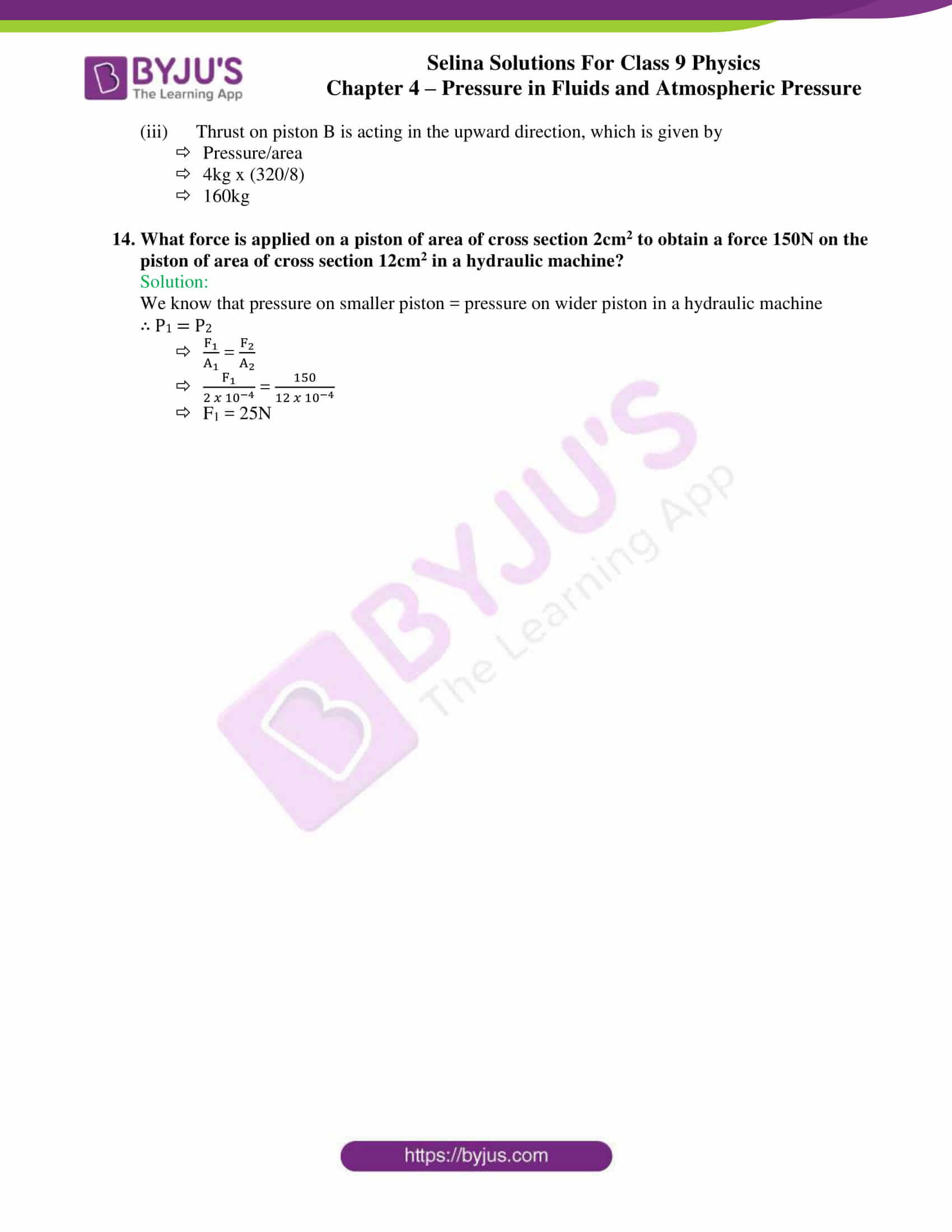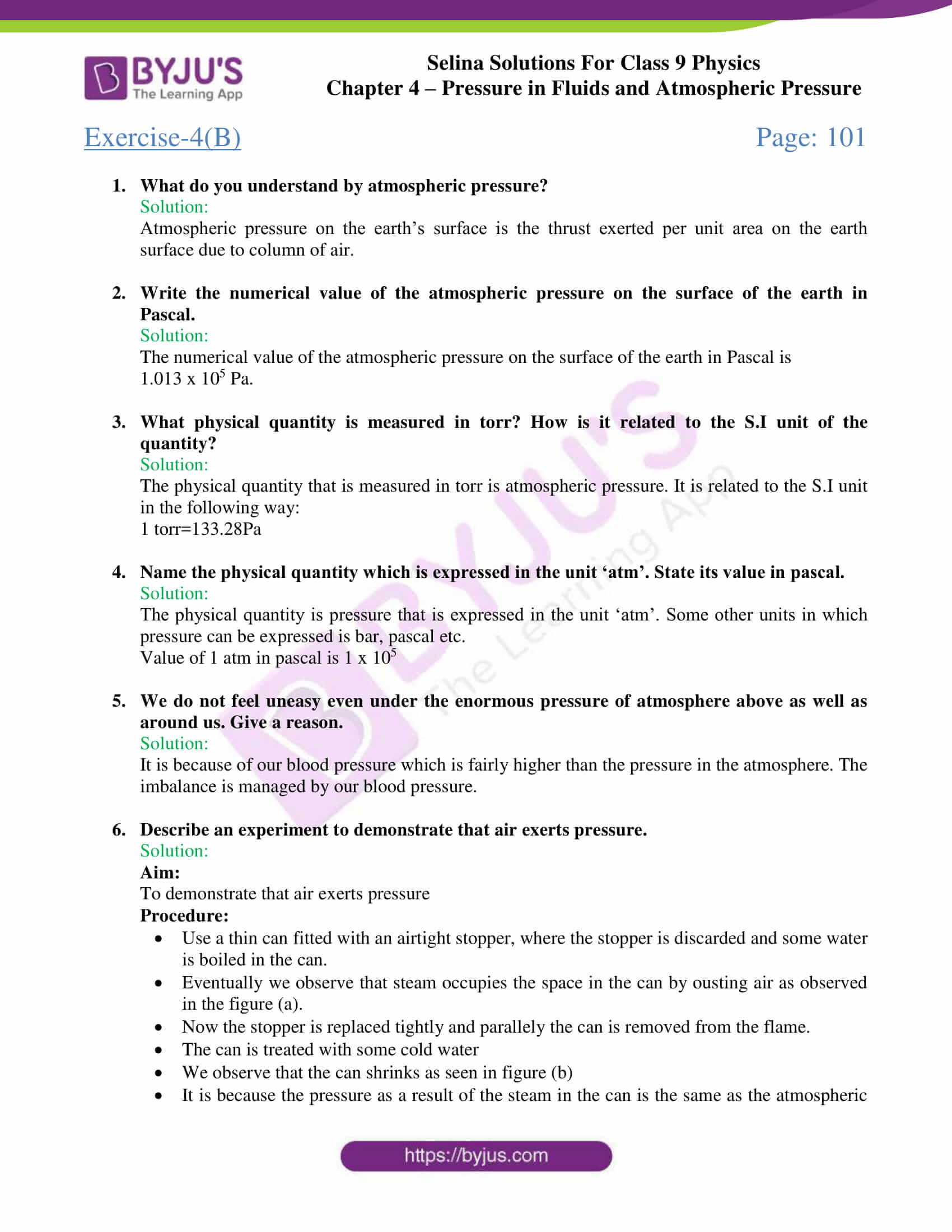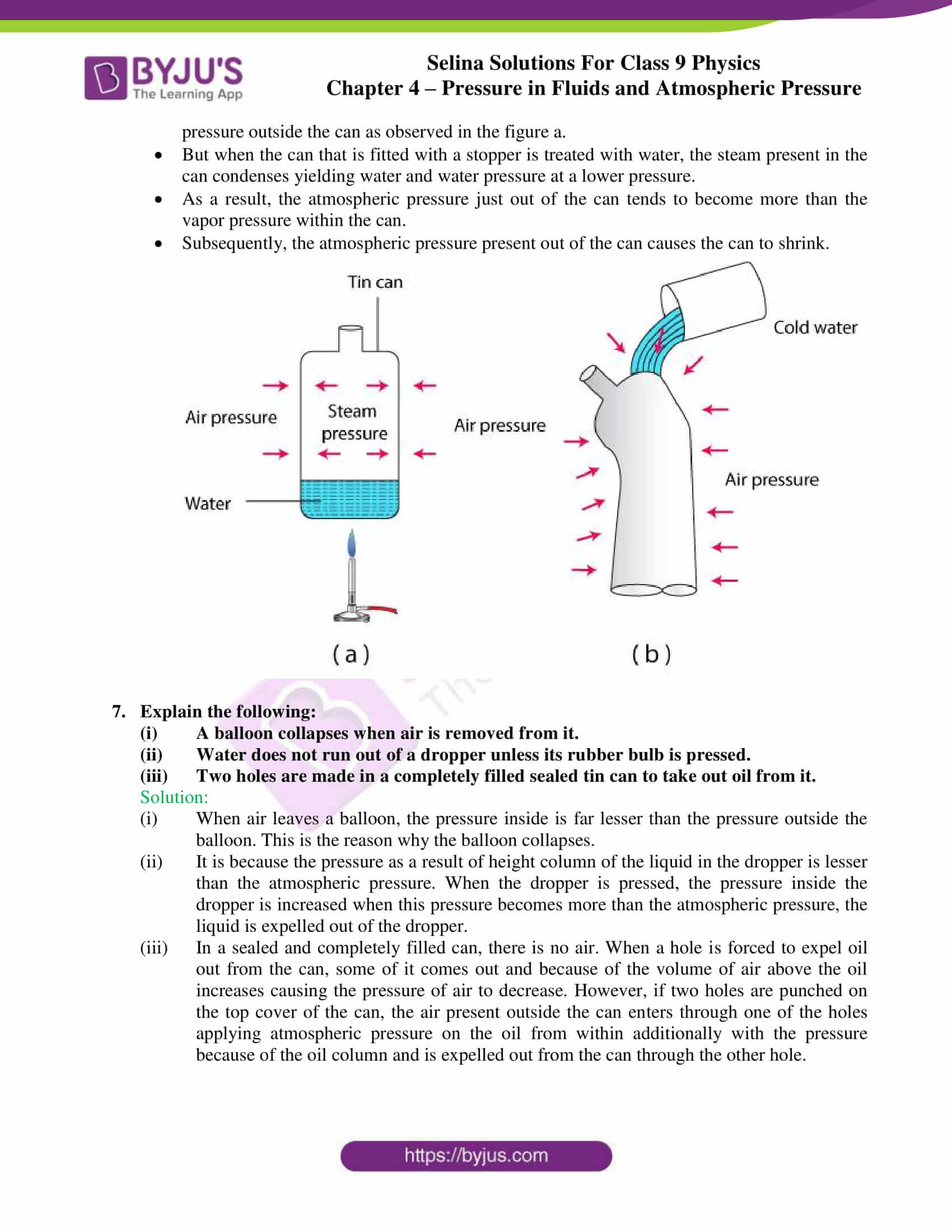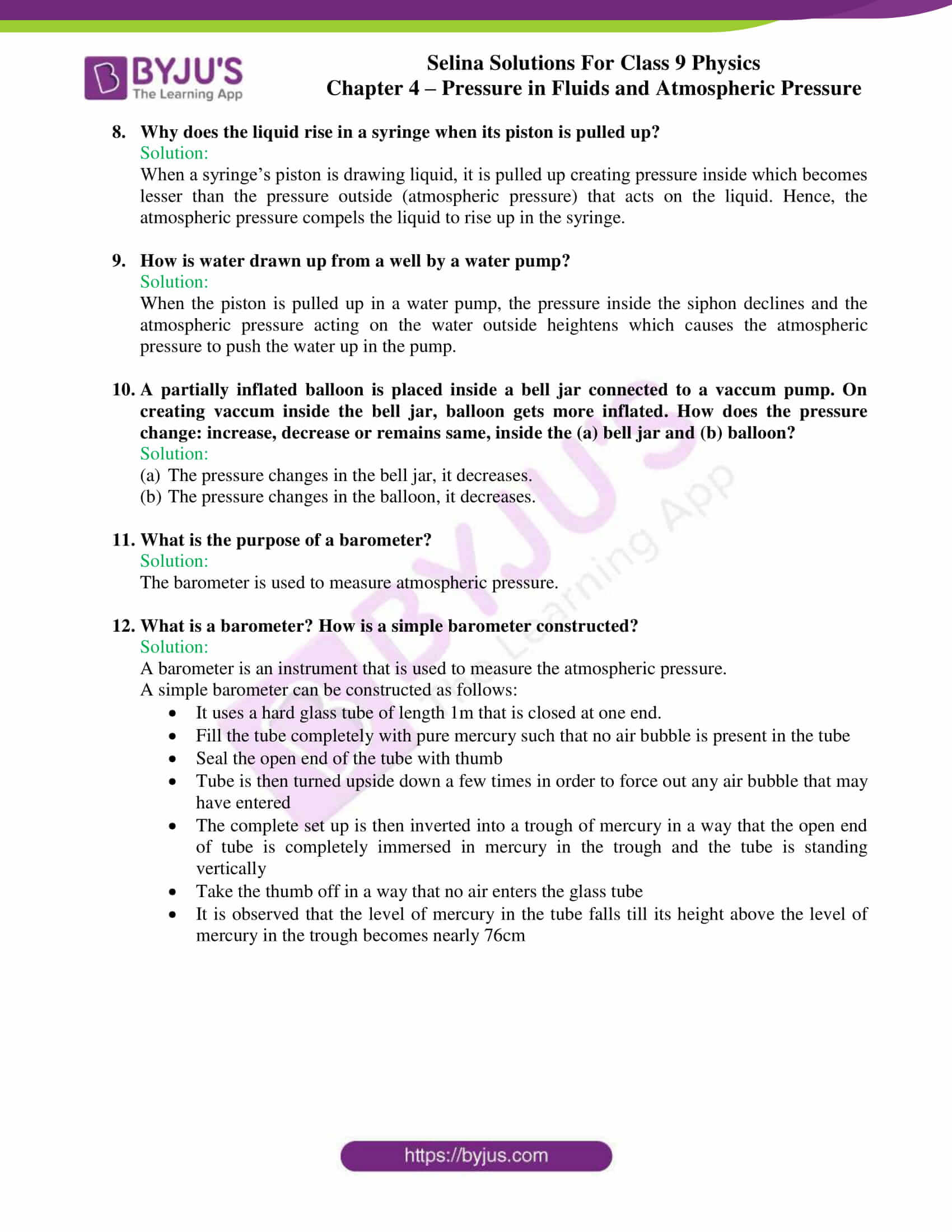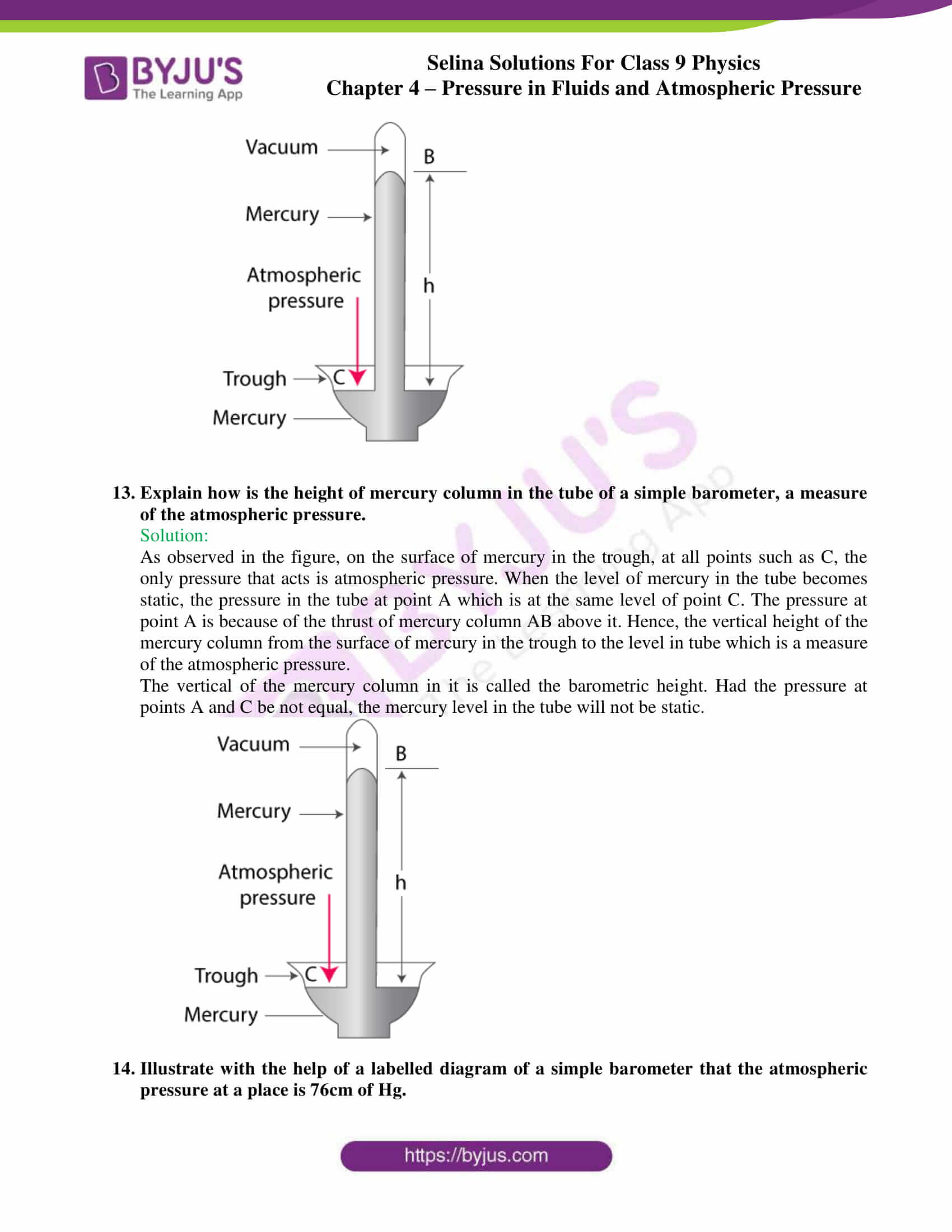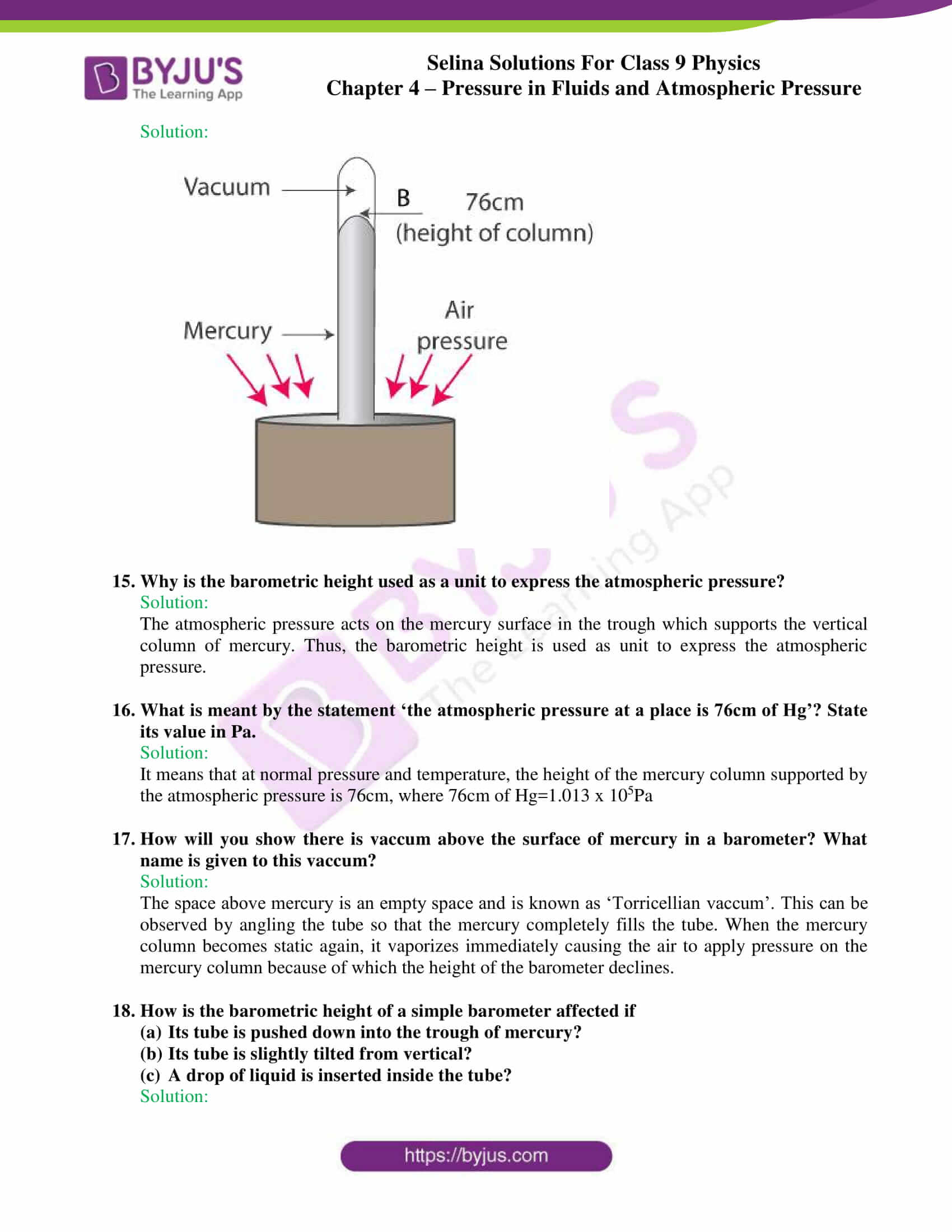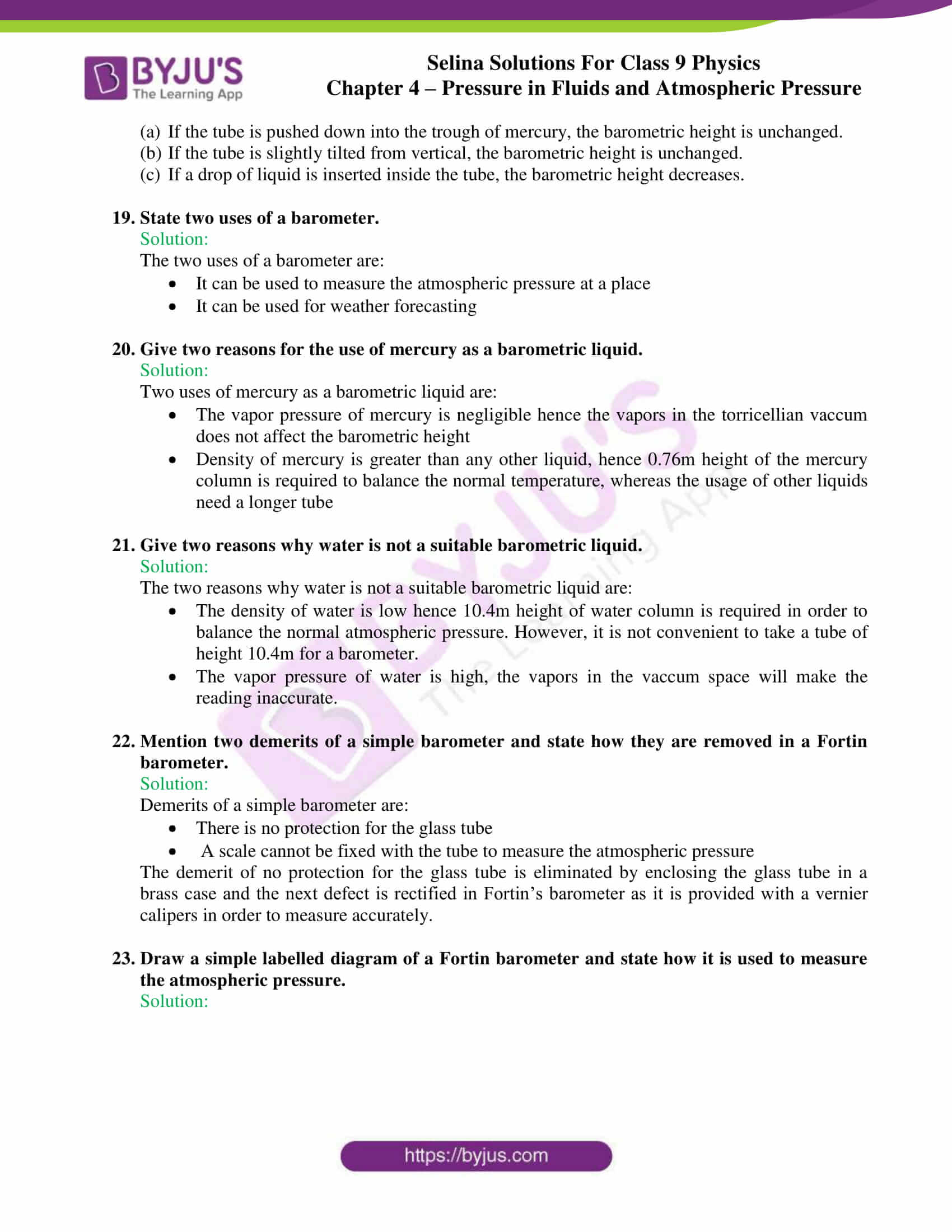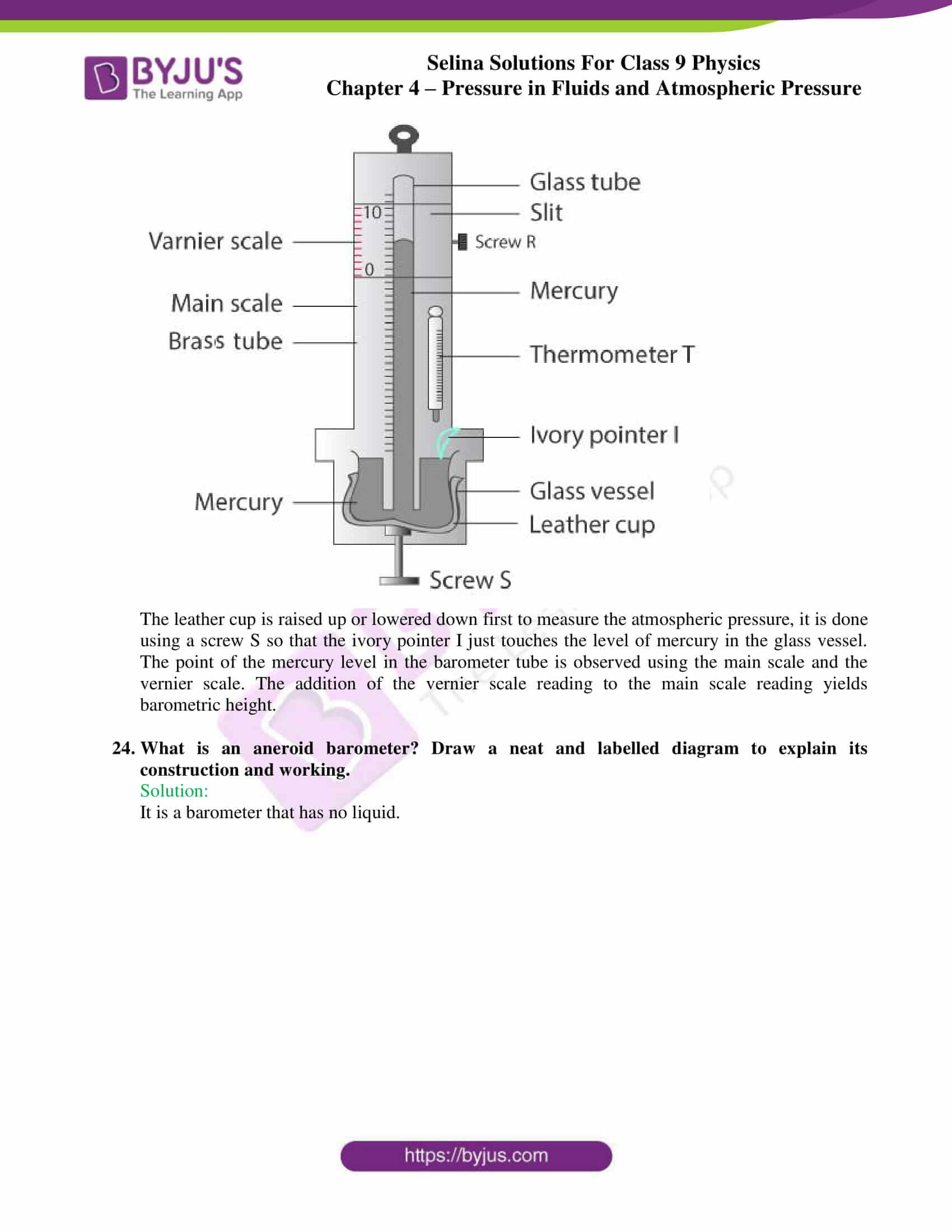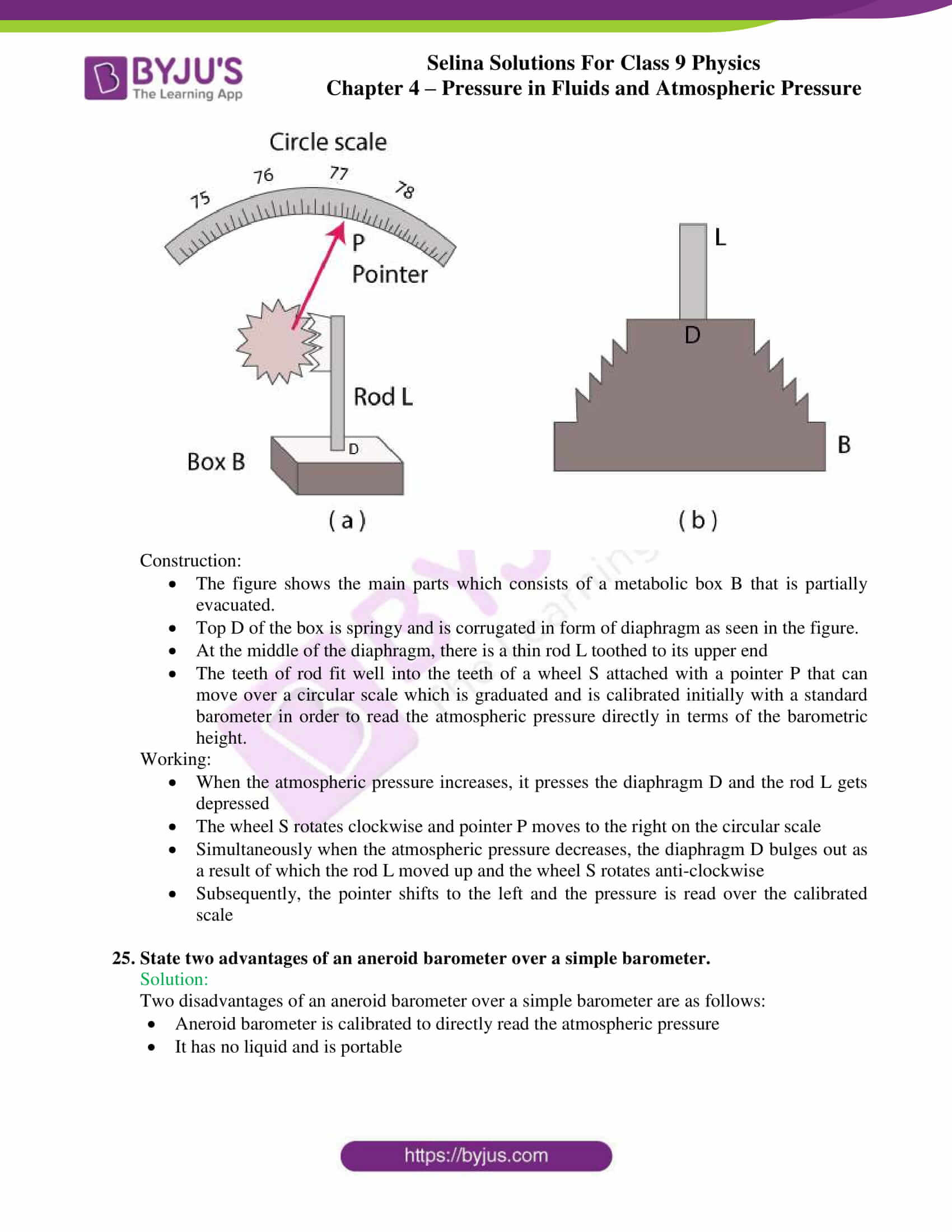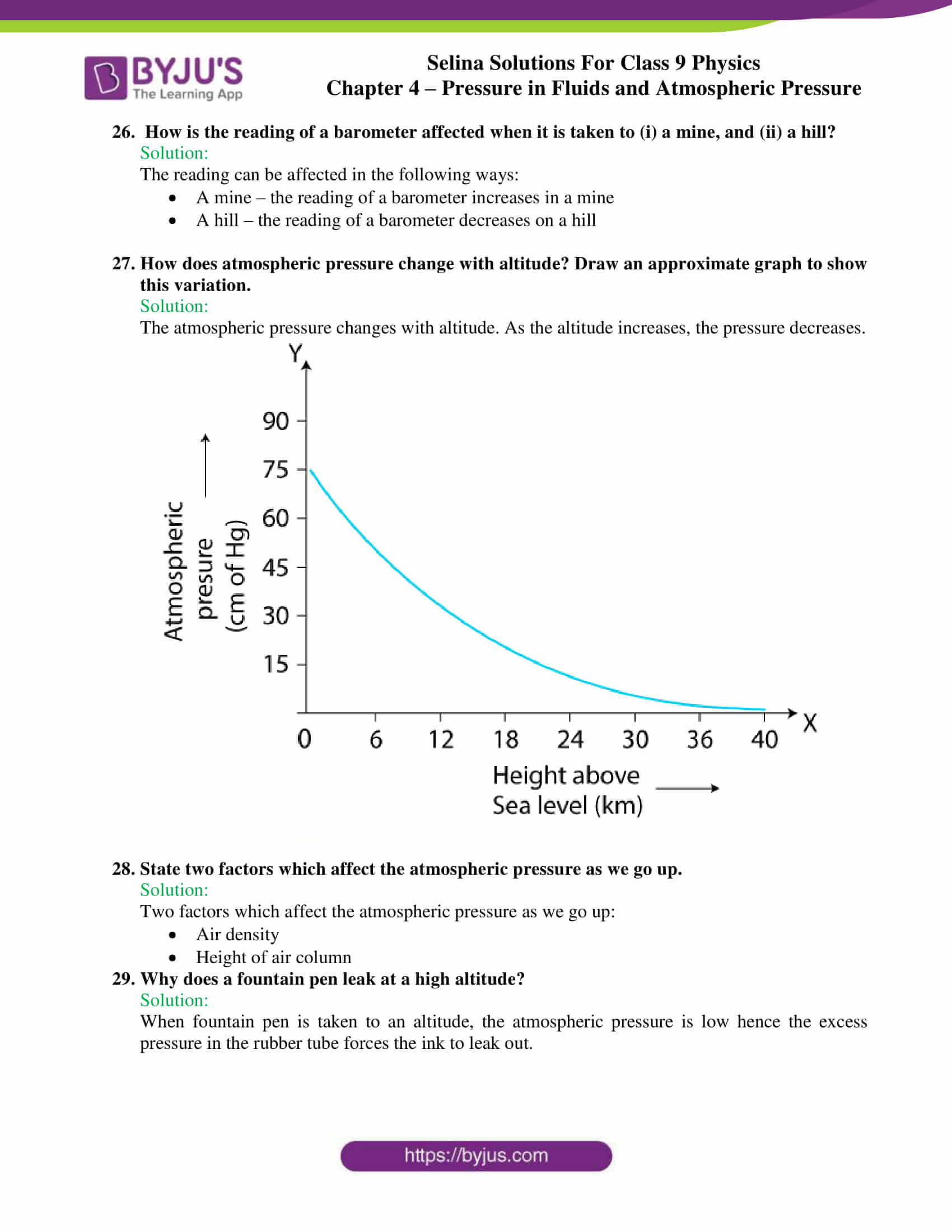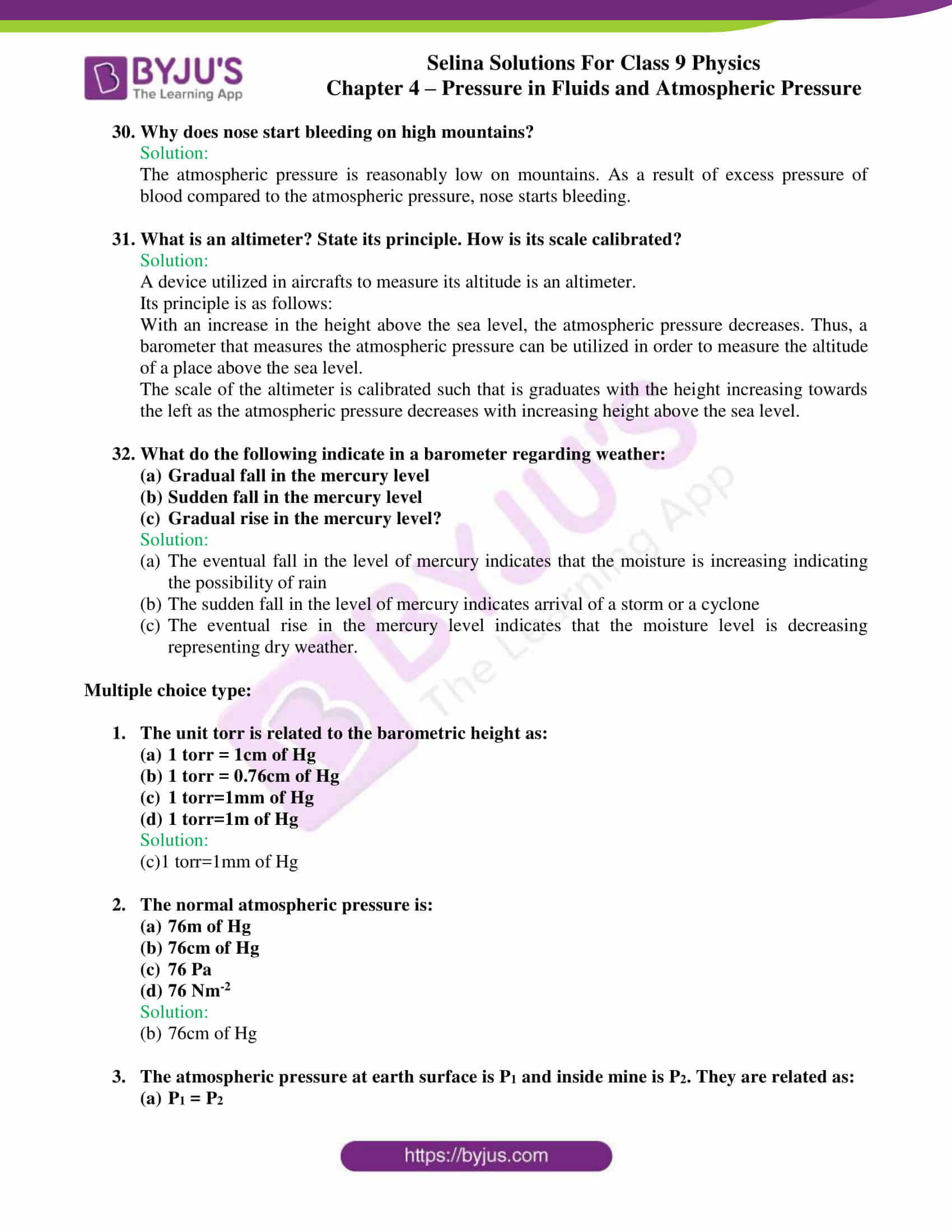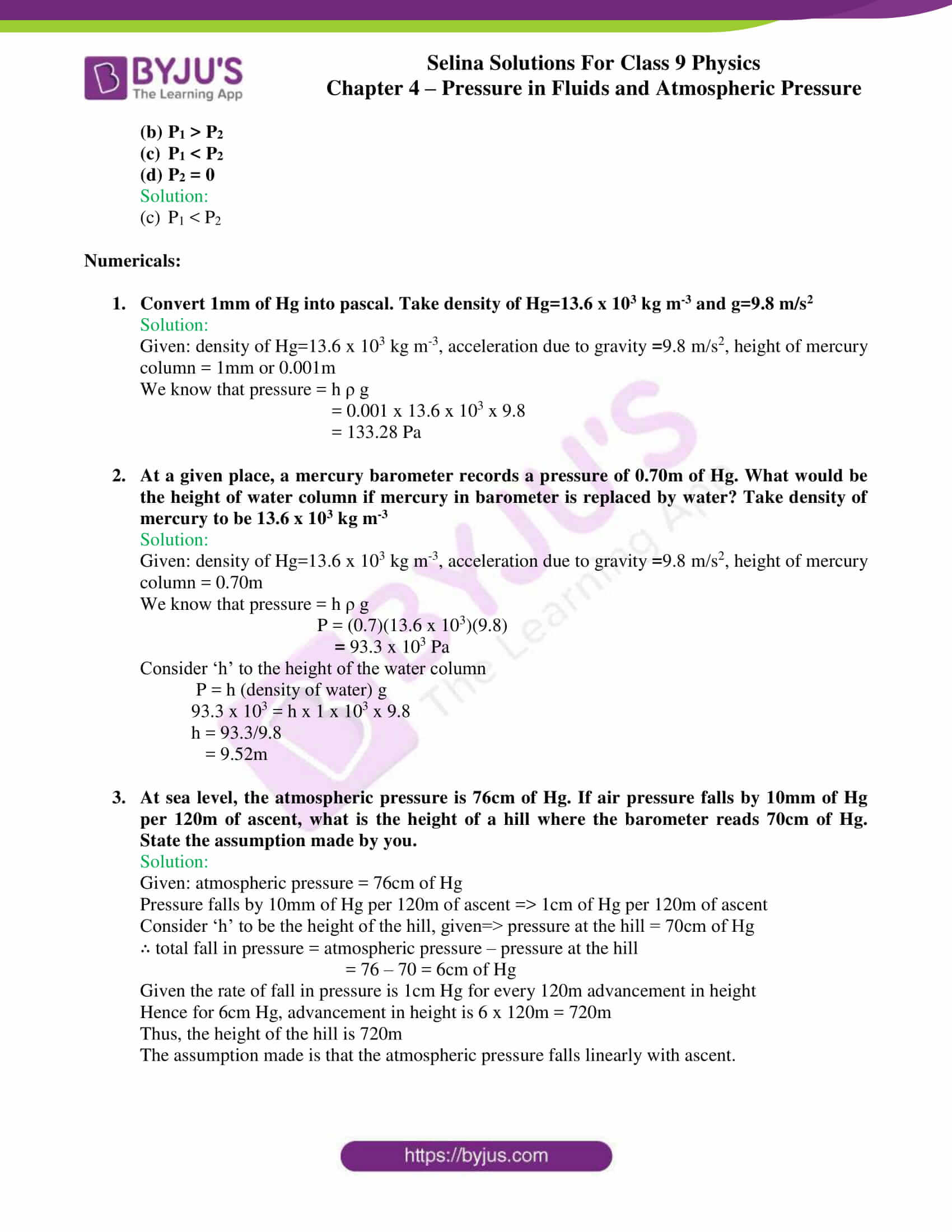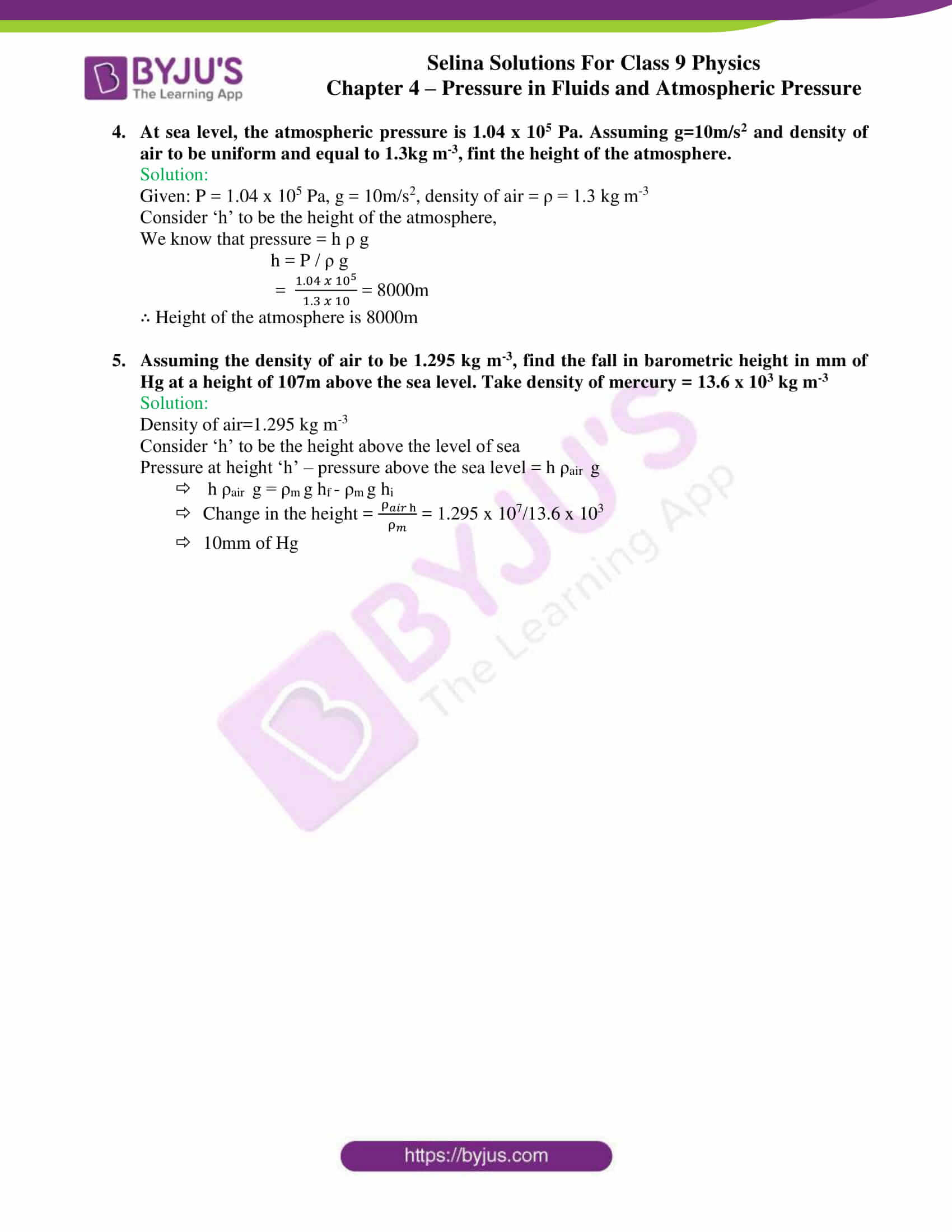## Selina Solutions for class 9 Physics Chapter 4 – Pressure in Fluids and Atmospheric Pressure

ICSE Class 9 Physics 4 – Pressure in Fluids and Atmospheric Pressure is covered as the fourth chapter in the ICSE physics textbook for Class 9 students. The chapter provides a detailed description of pressure in fluids and how they are transmitted across a medium.

Thrust and pressure are two important concepts that are introduced to students through this chapter. Along with the theoretical aspects, practical concepts are also covered by providing with engaging numerical problems towards the end of these concepts.

Students are also enlightened about Pascal’s law, its various applications such as – hydraulic press, hydraulic jack and hydraulic brakes along with examples. The envelope of air around the earth is called atmosphere, the weight of the air column exerts a thrust on the surface of the earth, the thrust exerted on unit area of earth’s surface is called atmospheric pressure.

List of subtopics covered in Chapter 4 – Pressure in Fluids and Atmospheric Pressure:

 Number Subtopic 4.1 Thrust and pressure 4.2 Pressure in fluids 4.3 The pressure exerted by a liquid column 4.4 Laws of liquid pressure 4.5 Some consequences of liquid pressure 4.6 Transmission of pressure in liquids, Pascal’s law 4.7 Application of Pascal’s Law 4.8 Examples of Hydraulic machines 4.9 Atmospheric pressure 4.10 Demonstration of atmospheric pressure 4.11 Common consequences of atmospheric pressure 4.12 Measurement of atmospheric pressure 4.13 Variation of atmospheric pressure with altitude 4.14 Weather forecast by the use of barometer 4.15 Altimeter

List of Exercise

 Name of the exercise Number of questions Page number Exercise-4A 51 93 Exercise-4B 40 101

## Selina Solutions for class 9 Physics Chapter 4 – Pressure in Fluids and Atmospheric Pressure

In this chapter, students are presented with a detailed explanation of pressure in fluids and the transmission of the pressure. Thrust and pressure are often co-related. Through this chapter, clear differences are set out for students to understand the differentiation.

Some other interesting concepts covered are the experimental demonstration of fluid pressure and its derivation, the laws of liquid pressure, some consequences of liquid pressure, Pascal’s law – transmission of pressure in liquids.

Pascal’s law has significance and has been applied in hydraulic jacks, hydraulic press and hydraulic brakes. The next interesting concept discussed is atmospheric pressure which is aided with a demonstration of atmospheric pressure through experiments provided in the textbook with labelled diagrams.

Also discussed are the various ways of measuring atmospheric pressure with the help of the following:

• Simple barometer
• Fortin’s barometer
• Aneroid barometer

### Key Features of Selina Solutions for class 9 Physics Chapter 4 – Pressure in Fluids and Atmospheric Pressure

• It is the best study tool that enables hassle-free preparation
• Ultimate solutions to textbook questions framed in accordance with the information provided in the textbook
• Solutions are explained with the help of labelled diagram wherever necessary
• Numericals are presented with detailed explanation with corresponding units
• Step by step solutions to all the physics concepts

## Frequently Asked Questions on Pressure in fluids and Atmospheric pressure

### Define the term thrust?

Thrust is the force acting normally on a surface. The S.I. unit of thrust is the newton(N).

### What is meant by pressure?

Pressure is the thrust per unit area of surface. The S.I. unit of pressure is newton per metre square

### Define one pascal?

One pascal can be defined as the pressure exerted on a surface of area 1m2 by a force of 1N that acts normally on it. The S.I. unit of pressure is newton per metre square

### Pressure is a scalar or vector?

Pressure is a scalar quantity.

### What is a fluid?

Any substance that can flow is known as a fluid.

### Name two applications of Pascal’s law?

Two applications of Pascal’s law are:

• Hydraulic press
• Hydraulic jack

### What do you understand by atmospheric pressure?

Atmospheric pressure on the earth’s surface is the thrust exerted per unit area on the earth surface due to the column of air.